Recursion is an important programming technique that lends itself to a variety of areas, not least of which is creating interesting visual images. In brief, recursion is a technique for breaking a complex problem into smaller and simpler versions of itself (this is called the “recursive step”) until the problem is trivial to solve (this trivial case is called the “base case”). This Codebox a variety of sketches that will help you explore this fun and interesting technique using simple geometrical concepts.

Many artists have used recursive themes in their work, particularly M.C. Escher and Rene Magritte. This first sketch shows how to create images in the style of Piet Mondrian, who is best known for his compositions of colorful rectangles.

The following program uses recursion to creates a faux-Mondrian. The base case is simple: all you do is draw a filled rectangle with a thick black border; the rectangle’s color is selected from a small set of primary colors (red, white, yellow, etc). The recursive step is to break this rectangle (and all subsequent rectangles) into 4 smaller rectangles by selecting a random point in its interior. The following figure should give you an idea of how it works: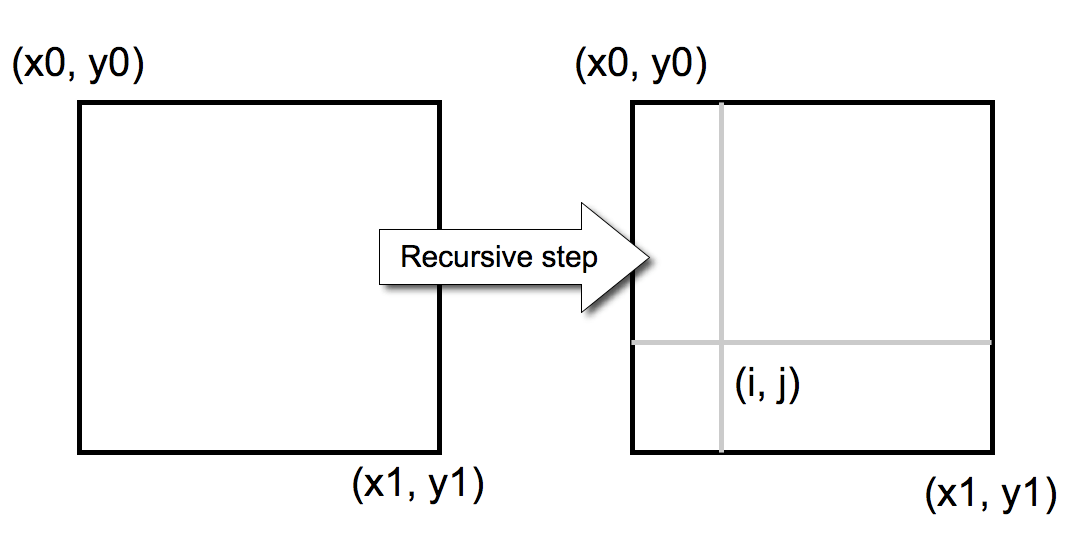The output will look something like this:Finally, here’s the sketch, mondrian.pde:

The piet() function is the heart of the program, and illustrates how most recursive programs work. Here it is:

``````
// Draw a Mondrian-inspired image using recursion
void piet(int x0, int y0, int x1, int y1, int N) {
if (N == 0) {
// Base case -- draw a colorful rectangle with a thick black border
int sw = 3; //this is the stroke width for the rectangle's border
color c[] = { #ff0000, #00ff00, #0000ff, #ffff00, #ffffff}; //Mondrian color palatte
fill(c[int(random(c.length))]);
strokeWeight(sw);
rect (x0,y0,x1-x0-sw,y1-y0-sw);
} else {
//Recursive step -- break the current rectangle into 4 new random rectangles
int i = int(random(x0,x1));
int j = int(random(y0,y1));
piet(x0,y0,i,j,N-1); // upper left rectangle
piet(i,y0,x1,j,N-1); // upper right rectangle
piet(x0,j,i,y1,N-1); // lower left rectangle
piet(i,j,x1,y1,N-1); // lower right rectangle
}
}
```
```

The function accepts 5 arguments: the coordinates of the upper left hand corner of a rectangle (x0 and y0), the coordinates of the lower right hand corner (x1 and x2), and the current recursive depth (N). The function does two basic things. First, it tests to see if it’s reached the “base case,” when N = 0. If so, then all it does is draw a rectangle that is randomly colored from the basic Mondrian palette of colors (red, greed, blue, yellow, and white). If it’s not the base case, then it selects a random point (i,j) inside the current rectangle, and then calls piet() 4 more times, passing in the various coordinates that form the 4 new interior rectangles. This “divide and conquer” approach of having a function that calls itself is the hallmark of recursion.

Simple recursive processes are often used to create natural looking objects. For example, we can create a natural looking plant using the following simple recursive process where each “plant” begins as a straight line. This line is replaced with a new “branch” that consists of the original line plus 3-4 new alternating branches. (The exact number of branches is determined by a variable you can set). This same process is applied recursively for each new branch, up to a depth you specify. The following figure illustrates the basic idea:Lines are represented as vectors. A vector consists of an origin point defined by the variables x and y, a length defined by the variable r, and an angle defined by the variable theta. The following figure shows how these variables are related: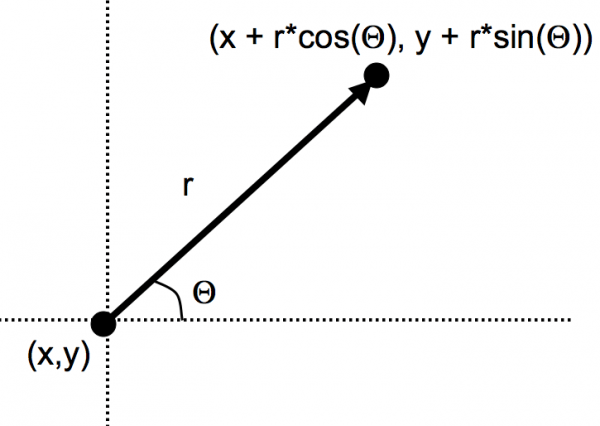This figure illustrates a few of the simple “plants” created with this basic process: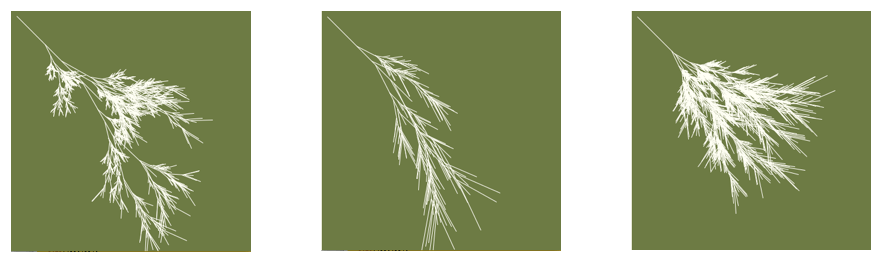Here’s the sketch, plant.pde:

Finally, I’d be remiss without showing the Snowflake curve, one of the best known recursive figures. The base case is a simple triangle. Each edge is then replaced by a new set of 4 line segments that are 1/3 the length of the original line. You then repeatedly replace each of these new segments with a new 4-line segment (this recursive step). The following figure should give you the basic idea of the process: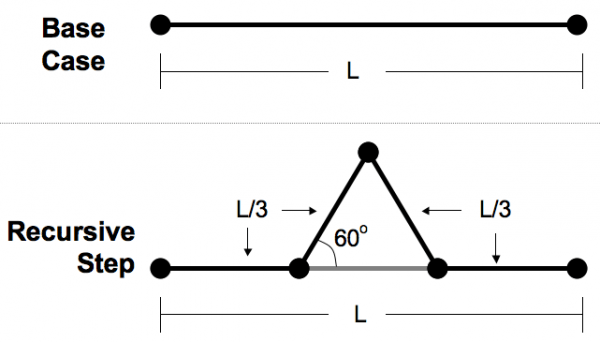The results look like this: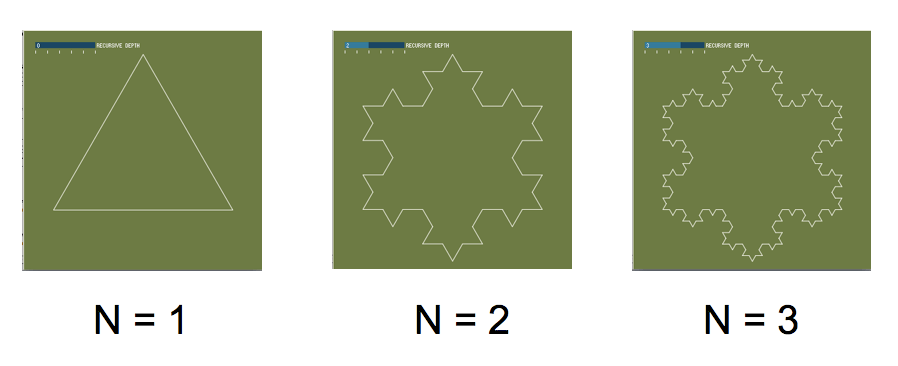Here’s the sketch, snowflake.pde. Note that you’ll need to have the controlP5 contributed library to change the recursive depth (often referred to as N) to see how it affects the image. (Unfortunately, I had to limit the maximum recursive depth to 6, rather than infinity, since the library does not currently support slidebars that extend beyond your monitor screen and into the far reaches of the known universe.)

More:
Check out all of the Codebox columns

In the Maker Shed:Getting Started with Processing
Learn computer programming the easy way with Processing, a simple language that lets you use code to create drawings, animation, and interactive graphics. Programming courses usually start with theory,but this book lets you jump right into creative and fun projects. It’s ideal for anyone who wants to learn basic programming, and serves as a simple introduction to graphics for people with some programming skills.

## 12 thoughts on “Codebox: Explore Recursion with Processing”

1.Bertrand Le Roy says:

Wow, Mondrian, folks, not Mondarian.

1.Andrew Odewahn says:

Yikes! Just fixed that.

2.Mahmoud says:

Dear Andrew Odewahn

How can I get a real world graph, for example Cairo(Egypt) , 2000 nodes, in a computer program?*I knew that, it is possible to generate a random graph with 2000 nodes with an arbitrary number of edges but this graph still unrealistic example,
I want to make a graph for an arbitrary city like Cairo, which have 2000 nodes and an arbitrary number of edges

I got a XML file by using JOSM.

Could you please help me to  know the answer of the question: How can I transform this XML file into a graph of nodes and edges( assgacency Matrex, or Incidence list )  I hope if you give me some keys to understand this pointRegards,I hope if you give me some keys to understand this point
Regards,Moussa
I got a XML file by using JOSM.

How can I transform this XML file into a graph of nodes and edges( assgacency Matrex, or Incidence list )

I hope if you give me some keys to understand this pointRegards,
I hope if you give me some keys to understand this pointRegards,
Moussa

3.Mahmoud says:

Dear Andrew Odewahn

How can I get a real world graph, for example Cairo(Egypt) , 2000 nodes, in a computer program?*I knew that, it is possible to generate a random graph with 2000 nodes with an arbitrary number of edges but this graph still unrealistic example,
I want to make a graph for an arbitrary city like Cairo, which have 2000 nodes and an arbitrary number of edges

I got a XML file by using JOSM.

Could you please help me to  know the answer of the question: How can I transform this XML file into a graph of nodes and edges( assgacency Matrex, or Incidence list )  I hope if you give me some keys to understand this pointRegards,I hope if you give me some keys to understand this point
Regards,Moussa
I got a XML file by using JOSM.

How can I transform this XML file into a graph of nodes and edges( assgacency Matrex, or Incidence list )

I hope if you give me some keys to understand this pointRegards,
I hope if you give me some keys to understand this pointRegards,
Moussa

4.ツイード ダウン says:

クラークスのデザートブーツ ツイード ダウン http://wanjiebuz.ferragamobyhidejp.org/